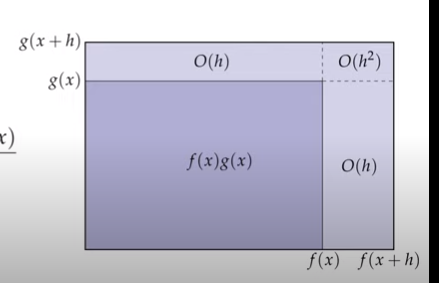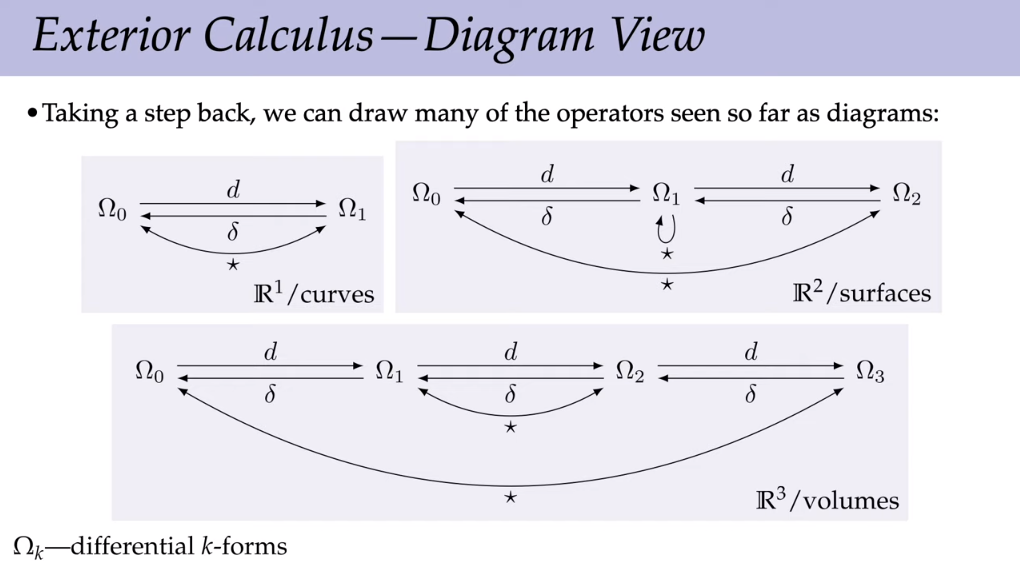# DDG Lecture 6

## Exterior Derivative

two big ideas in calculus: differentiation vs. integration. linked by fundamental theorem of calculus.

Exterior calculus:

• differentiation of k-forms (exterior derivative)
• integration of k-forms (measure volume)
• linked by Stoke's Theorem

Goal: integrate over meshes to get discrete exterior calculus.

### Motivation for exterior calculus.

Why generalize vector calculus?

• Hard to measure change in volume
• Duality clarifies distinction between different concepts/quantities.
• Topology: notion of differentiation doesn't require metric (e.g. cohomology)
• Geometry: clear language for calculus on curved surfaces.
• Physics: clear distinction between physical quantities (e.g. velocity vs. momentum)
• CS: leads directly to discretization

### Exterior Derivative

Derivative as limit, slope, tangent plane, "best linear approximation".

Vector derivatives: gradient $R \rightarrow R^n$, divergence $R^n \rightarrow R$, curl $R^n \rightarrow R^n$

In coordinates:

$\phi : \mathbb{R}^n \rightarrow \mathbb{R}$

$X = u \frac{\partial}{\partial x} + v \frac{\partial}{\partial y} + w \frac{\partial}{\partial z}$ where $u,v,w : \mathbb{R^n} \rightarrow \mathbb{R}$ are coordinate functions and $\frac{\partial}{\partial x}, \frac{\partial}{\partial y}, \frac{\partial}{\partial z}$ are the basis vector fields.

defn:

grad

$\nabla \phi = \frac{\partial \phi}{\partial x}\frac{\partial}{\partial x} + \frac{\partial \phi}{\partial y} \frac{\partial}{\partial y} + \frac{\partial \phi}{\partial z} \frac{\partial}{\partial z}$

div

$\nabla \cdot X = \frac{\partial u}{\partial x} + \frac{\partial v}{\partial y} + \frac{\partial w}{\partial z}$

curl

\begin{aligned} \nabla \times & X = \\ & \left( \frac{\partial w}{\partial y} - \frac{\partial v}{\partial z} \right) \frac{\partial}{\partial x} + \\ & \left( \frac{\partial u}{\partial z} - \frac{\partial w}{\partial x} \right) \frac{\partial}{\partial y} + \\ & \left( \frac{\partial v}{\partial x} - \frac{\partial u}{\partial y} \right) \frac{\partial}{\partial z} \end{aligned}

#### The Exterior Derivative

Let $\Omega^k$ denote the space of all differential k-forms.

Unique linear map $d: \Omega^k \rightarrow \Omega^{k+1}$ such that

• differential : for k=0, $d \phi(X) = D_X \phi$
• take the "directional derivative of $\phi$ along $X$
• product rule : $d(\alpha \wedge \beta) = d \alpha \wedge \beta + (-1)^k \alpha \wedge d \beta$
• exactness : $d \circ d = 0$

## Exterior Derivative -- Differential

review: Directional Derivative

defn The directional derivative of a scalar function $\phi$ at a point $p$ with respect to a vector $X$ is the rate at which that function increases as we walk away from $p$ with velocity $X$.

$D_X \phi |_p := \lim_{\epsilon \rightarrow 0} \frac{\phi(p+\epsilon X) - \phi(p)}{\epsilon}$

This is trivially extended to $X$ vector field instead of at point $p$ by applying previous definition pointwise. The result is a scalar function.

Gradient:

$\left< \nabla \phi, X \right> = D_X \phi ~ \forall X$

i.e the gradient is the unique vector field $\nabla \phi$ whose inner product with any vector field X yields the directional derivative along X. (Note: depends on defn of inner product.)

### Differential of a function

0-forms are scalar functions. Change in a scalar function can be measured via the differential. Two ways to define differential:

1. As unique 1-form such that applying any vector field gives directional derivative along those directions.
- $d\phi(X) = D_X \phi$

1. In coordinates:
- $d \phi := \frac{\partial \phi}{\partial x^1} dx^1 + \cdots + \frac{\partial \phi}{\partial x^n} dx^n$


Conceptually similar to the gradient.

graddifferential
$\left< \nabla \phi \right> = D_X \phi$$d \phi(X) = D_X\phi$

what's the difference?

• type: vector field vs. differential 1-form
• dependence on inner product.
• $d\phi(\cdot) = \left<\nabla \phi, \cdot \right>$
• we have no dependence on geometry for differential.
$(d\phi)^\sharp = \nabla \phi \iff d\phi(\cdot) = \left<\nabla \phi, \cdot \right> \iff (\nabla \phi)^\flat = d\phi$

## Exterior Derivative -- Product Rule

Q: why is it true that ab = ba for a,b in R rectangle area implies commutativity.

Preduct rule of differentiation of real functions.(We neglect the O(n^2) term as differentiation is linear approximation on crack.)

let $\alpha$ be a k-form and $\beta$ be an l-form. Then

$d(\alpha \wedge \beta) = (d \alpha) \wedge \beta + (-1)^k \alpha \wedge (d\beta)$

### Recursive evaluation of differential form

Ex. Let $\alpha := u dx, ~ \beta := v dy, ~ \gamma := w dz$ be differential 1-forms on $\mathbb{R}^n$.

where $u,v,w : \mathbb{R}^n \rightarrow \mathbb{R}$ are 0-forms. Also, let $\omega := \alpha \wedge \beta$. Then,

\begin{aligned} d(\omega \wedge \gamma) &= (d\omega) \wedge \gamma + (-1)^2 \omega \wedge (d\gamma) \\ &= \left[ (d\alpha) \wedge \beta - \alpha \wedge (d\beta) \right] \wedge \gamma + (-1)^2 \omega \wedge (d\gamma) \\ &= (d\alpha) \wedge \beta \wedge \gamma - \alpha \wedge (d \beta) \wedge \gamma + \alpha \wedge \beta \wedge (d \gamma) \end{aligned}

Note,

$d\alpha = (du) \wedge dx + u (ddx)$

Therefore,

\begin{aligned} d(\omega \wedge \gamma) &= (d\alpha) \wedge \beta \wedge \gamma - \alpha \wedge (d \beta) \wedge \gamma + \alpha \wedge \beta \wedge (d \gamma) \\ &= (du \wedge dx) \wedge \beta \wedge \gamma - \alpha \wedge (dv \wedge dy) \wedge \gamma + \alpha \wedge \beta \wedge (dw \wedge dz) \end{aligned}

Ex. Let $\phi(x,y) := \frac{1}{2} e^{-(x^2+y^2)}$. Then

$d\phi = \frac{\partial \phi}{\partial x}dx + \frac{\partial \phi}{\partial y}dy \\ = -2 \phi(xdx + ydy)$

Ex. Let $\alpha(x,y) = xdx + ydy$ . Then.

\begin{aligned} d\alpha &= \left( \frac{\partial x}{\partial x}dx + \frac{\partial x}{\partial y}dy \right) \wedge dx + \left( \frac{\partial y}{\partial x} + \frac{\partial y}{\partial y} \right) dy \\ &= dx \wedge dx + dy \wedge dy \\ &= 0 \end{aligned}

Ex. Let $\alpha$ as above, then $d \star \alpha$

\begin{aligned} d \star \alpha &= d(\star(xdx + ydy)) \\ &= d(xdy - ydx) \\ &= dx \wedge dy - dy \wedge dx \\ &= 2 (dx \wedge dy) \end{aligned}

## Exterior Derivative -- Exactness

$d \circ d = 0$

Q: let $\alpha = udx + vdy + wdz$ where $u,v,w : \mathbb{R}^3 \rightarrow \mathbb{R}$. What is $d\alpha$? A:

\begin{aligned} d\alpha &= d(udx + vdy + wdz) = du \wedge dx + u \wedge d(dx) + dv \wedge dy + v \wedge d(dv) + dw \wedge dz + w \wedge d(dz) \\ &= du \wedge dx + dv \wedge dy + dw \wedge dz \\ &= \frac{\partial u}{\partial y}(dy \wedge dx) + \frac{\partial u}{\partial z}(dz \wedge dx) + \frac{\partial v}{\partial x}(dx \wedge dy) + \frac{\partial v}{\partial z}(dz \wedge dy) + \frac{\partial w}{\partial x}(dx \wedge dz) + \frac{\partial w}{\partial y}(dy \wedge dz) \\ &= \left(\frac{\partial v}{\partial x} - \frac{\partial u}{\partial y} \right) dx \wedge dy + \left(\frac{\partial w}{\partial y} - \frac{\partial v}{\partial z} \right) dy \wedge dz + \left(\frac{\partial u}{\partial z} - \frac{\partial w}{\partial x} \right) dz \wedge dx \end{aligned}

Vaguely reminiscent of the cross product. Thus reminiscent of curl.

### Curl

Let $X := u \frac{\partial}{\partial x} + v \frac{\partial}{\partial y} + w \frac{\partial}{\partial z}$.

Then

\begin{aligned} \nabla \times X \cong d \alpha = \\ & \left(\frac{\partial v}{\partial x} - \frac{\partial u}{\partial y} \right) dx \wedge dy \\ & + \left(\frac{\partial w}{\partial y} - \frac{\partial v}{\partial z} \right) dy \wedge dz \\ & + \left(\frac{\partial u}{\partial z} - \frac{\partial w}{\partial x} \right) dz \wedge dx \end{aligned}

In other words, $\nabla \times X = (\star dX^\flat)^\sharp$

### Divergence

Ex. Let ambient space be $\mathbb{R}^3$. Let $\alpha$ as before. What is $d \star \alpha$?

\begin{aligned} d \star \alpha &= d (\star(u dx + v dy + wdz)) \\ &= d(u dy\wedge dz + v dz \wedge dx + w dx \wedge dy) \\ &= du \wedge dy \wedge dz + dv \wedge dz \wedge dx + dw \wedge dx \wedge dy \\ &= \frac{\partial u}{\partial x} dx \wedge dy \wedge dz + \frac{\partial v}{\partial y} dy \wedge dz \wedge dx + \frac{\partial w}{\partial z} dz \wedge dx \wedge dy \\ &= \left( \frac{\partial u}{\partial x} + \frac{\partial v}{\partial y} + \frac{\partial w}{\partial z} \right) dx \wedge dy \wedge dz \end{aligned}

Vaguely reminiscent to the dot product or the divergence.

i.e $\nabla \cdot X = \star (d \star X^\flat)$

Define the codifferential $\delta := \star d \star$.

## Exterior vs. Vector Derivatives

graddivcurl
grad$\phi$div $X$curl $Y$
$(d\phi)^\sharp$$\star d (\star X^\flat)$$(\star(d Y^\flat))^\sharp$### Laplacian

$\Delta = \nabla \cdot \nabla$

or:

$\Delta = \star d \star d$

We can generalize to k-forms:

$\Delta := \star d \star d + d \star d \star$